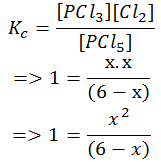# Calculating Equilibrium Concentrations

In a chemical reaction, when both the reactants and the products are in a concentration which does not change with time any more, it is said to be in a state of chemical equilibrium. In this state, the rate of forward reaction is same as the rate of backward reaction. For a reaction, if you know the initial concentrations of the substances, you can calculate the equilibrium concentration. Let us see how we do it with the help of an example.

## Steps to Calculate Equilibrium Concentration

Problem Statement: At 300K, 6.00 moles of PCl5 kept in 1 L closed reaction vessel was allowed to attain equilibrium. You are required to find the composition of the mixture at equilibrium. Given that Kc for the reaction is 1.

• Step1: Write the balanced equation for the reaction for which the concentration is to be calculated.

PCl5⇌ PCl3 + Cl2

• Step 2: Convert the given concentrations into Molarity. Here the amount of PCl5 before the reaction is 6 moles and the volume of the reaction vessel is 1 L. Therefore, the concentration of PCl5 is 6/1 moles/litre = 6 M.
• Step 3: Make a note of the initial concentration and the change in concentration for each substance on undergoing equilibrium. Change in concentration is calculated by taking x as the concentration of one of the reactants and then finding out the concentrations of other substances in terms of x.• Step 4: Using the Kc provided in the problem statement, fill in the values of the equilibrium concentrations in the equation. Note: While writing concentrations at equilibrium in the equation below, only those substances are taken into consideration whose concentrations change considerably.• Step 5: Solve for x. Since the concentration value cannot be negative, we take up the positive value of x. In other words, the value of x that makes chemical sense is taken.
x2+ x -6 = 0
The equation gives x = 2 or x = -3.
• Step 6: Calculate the values of equilibrium concentration for each substance using the value of x.
Thus, we have
[PCl5] = 6 – x = 6 – 2 = 4 M
[PCl3] = [Cl2] = x = 2 M

## Introduction to Chemical Equilibrium